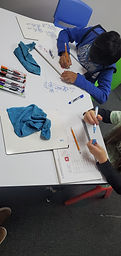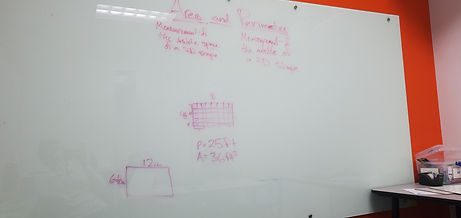Mr. Jake

Target 1​

Lesson Type:

Review

Measurment

:

Techniques and Formulas

Solve problems involving area.

1:

Recognize area as a measurement of 2D shapes.

2:

Recognize that area is measured in squared units (i.e., cm2), depicting that the measurement has both height and width.

3:

Find the area of composite figures.

4:

Decompose a composite figure into simple rectangles to compute area.

5:

Solve real-world area problems.

6th

Vocabulary:

Area, Perimeter, Composite

Activities:

Solved problems involving area and perimeter of composite shapes

Created shapes using given measurements

Discussed methods for finding area of composite shapesHome Exploration

Guiding Questions:Absent Students:

Target 2

:

1:

Define PEMDAS – Parenthesis, Exponents, Multiplication, Division, Addition, Subtraction.

2:

Correctly identify the order of operations and use them to simplify expressions.

3:

Understand that calculating in the wrong order can result in a wrong answer.

4:

Understand that the order of operations must be followed to evaluate expressions.

5:

Solve problems that have more than one operation.

7th

Vocabulary:

Order of Operations. PEMDAS

Activities:

Determined the answer of arithmetic problems that use more than one operationsHome Exploration

Guiding Questions:Target 3

:

Vocabulary:

Activities:Home Exploration